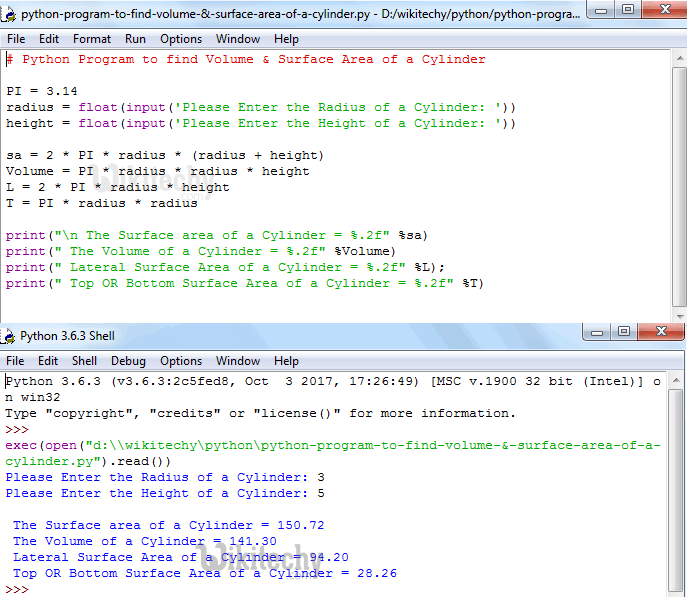# python tutorial - Python Program to find Volume & Surface Area of a Cylinder - learn python - python programming

• To write Python Program to find Volume and Surface Area of a Cylinder with example.
• Before we step into the program, Let see the definitions and formulas behind Surface Area of a Cylinder, Lateral Surface Area, Top or Bottom Surface Area and Volume of a Cylinder.Learn Python - Python tutorial - Python Program to find Volume & Surface Area of a Cylinder - Python examples - Python programs

## Surface Area of a Cylinder

• If we know the radius and height of the cylinder then we can calculate the surface area of a cylinder using the formula:

## Volume of a Cylinder

• The amount of space inside the Cylinder is called as Volume. If we know the height of a cylinder then we can calculate the Volume of a cylinder using formula:

## Python Program to find Volume & Surface Area of a Cylinder

• This Python program allows the user to enter the value of a radius and height.
• Using these values, it will calculate the Volume of a Cylinder, Surface Area of a Cylinder, Lateral Surface Area of a Cylinder, Top Or Bottom Surface Area of a Cylinder as per the formula.

## Analysis

• First, We declared PI variable and assigned the value as 3.14.
• Below statements will ask the user to enter radius and height values and it will assign the user input values to respected variables. Such as first value will be assigned to radius and second value to height
• Next, We are calculating Volume, Surface Area, Lateral Surface Area, Top Or Bottom Surface Area of a Cylinder using their respective Formulas:
• Following print statements will help us to print the Volume and Surface area of a Cylinder

## OutputLearn Python - Python tutorial - Python Program to find Volume & Surface Area of a Cylinder - Python examples - Python programs

• We have entered the radius of a Cylinder = 3 and height = 5

The Surface Area of a Cylinder is
Surface Area of a Cylinder= 2πr² + 2πrh
It can also be written as
Surface Area of a Cylinder = 2πr (r+h)
Surface Area of a Cylinder = 2 * PI * radius * (radius + height)
Surface Area of a Cylinder = 2 * 3.14 * 3 * (3+5);
Surface Area of a Cylinder = 150.72

The Volume of a Cylinder is
Volume of a Cylinder = πr²h
Volume of a Cylinder = PI * radius * radius * height
Volume of a Cylinder = 3.14 * 3 * 3 * 5
Volume of a Cylinder = 141.3

The Lateral Surface Area of a Cylinder is
L = 2πrh
L = 2 * PI * radius * height
L = 2 * 3.14 * 3 * 5
L = 94.2

The Top Or Bottom Surface Area of a Cylinder is
T = πr²
T = 3.14 * 3 * 3
T = 28.26

NOTE: For the calculation purpose we have taken π = 3.14 instead of (3.142857142..). So, All the above values are almost equal to program output but may differ at 0.01.

## Python Program to find Volume & Surface Area of a Cylinder using functions

• This program allows user to enter the value of a radius and height.
• We will pass the radius value to the function argument and then it will calculate the Volume of a Cylinder, Surface Area of a Cylinder, Lateral Surface Area of a Cylinder, Top Or Bottom Surface Area of a Cylinder as per the formula.

## OutputLearn Python - Python tutorial - Python Program to find Volume & Surface Area of a Cylinder using functions - Python examples - Python programs

## Analysis

• First, We imported the math library using the following statement.
• This will allow us to use the mathematical functions like math.pi.
• If you fail to include this line then math.pi will through an error.
• We defined the function with two argument using def keyword. It means, User will enter the radius and height of a Cylinder.
• We are calculating Volume, Surface Area, Lateral Surface Area, Top Or Bottom Surface Area of a Cylinder as we explained in first example

NOTE: We can call the function with arguments in .py file directly or else we can call it from the python shell. Please don’t forget the function arguments